# if else statement in java

#### View more Tutorials: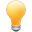Follow us on our fanpages to receive notifications every time there are new articles.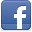Facebook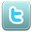Twitter

## 1- if statment

The if statement is the most basic of all the control flow statements. It tells your program to execute a certain section of code only if a particular test evaluates to true.
Below is the structure of if statement:
``````

if (condition)  {
// Do something here if 'condition' is true.
}
``````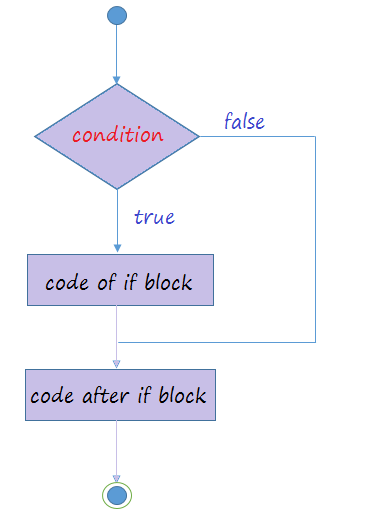The above image illustrates the flow of application when it meets a if statement.
1. The program checks the condition.
2. If the condition is true, code in the if block will be executed. It then continues executing the codes below the if block.
3. If the condition is false, the program will ignores the code in the if block and execute code snippets below the if block​​​​​​​.
Example:
IfExample.java
``````
package org.o7planning.tutorial.javabasic.controlflow;

public class IfExample {

public static void main(String[] args) {

// Declare a variable, representing your age
int age = 30;

System.out.println("Your age: " + age);

// The condition to test is 'age> 20'
if (age > 20) {

System.out.println("Okey!");
System.out.println("Age is greater than 20");
}

// The code after the 'if' block.
System.out.println("Done!");

}

}
``````
Run the example:
``````
Okey!
Age is greater than 20
Done!
``````

## 2- if - else statement

The if-else statement also tests the condition. It executes the if block if condition is true otherwise else block is executed.
** if - else **
``````
if( condition )  {
// Do something here
}
// Else
else  {
// Do something here
}
``````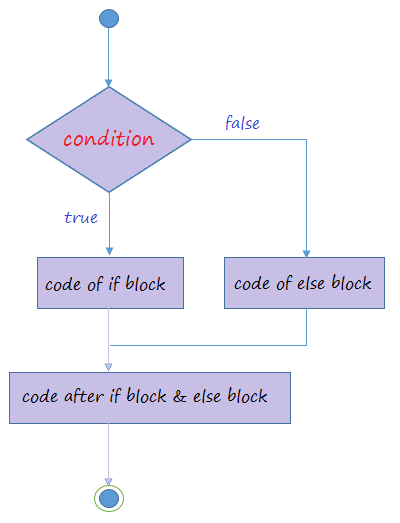Example:
IfElseExample.java
``````
package org.o7planning.tutorial.javabasic.controlflow;

public class IfElseExample {

public static void main(String[] args) {

// Declare a variable, representing your age
int age = 15;

System.out.println("Your age: " + age);

// The condition to test is 'age> 18'
if (age >= 18) {

System.out.println("Okey!");
System.out.println("You are accepted!");

} else {

System.out.println("Sorry!");
System.out.println("Age is less than 18");
}

// The code after the 'if' block and 'else' block.
System.out.println("Done!");

}

}
``````
Running the example:
``````
Sorry!
Age is less than 18
Done!
``````

## 3- if - else if - else statement

The structure of an if - else if - else statement is:
``````
if(condition 1)  {

// Do something here
} else if(condition 2) {

// Do something here
} else if(condition 3) {

// Do something here
}
// Else
else  {

// Do something here
}
``````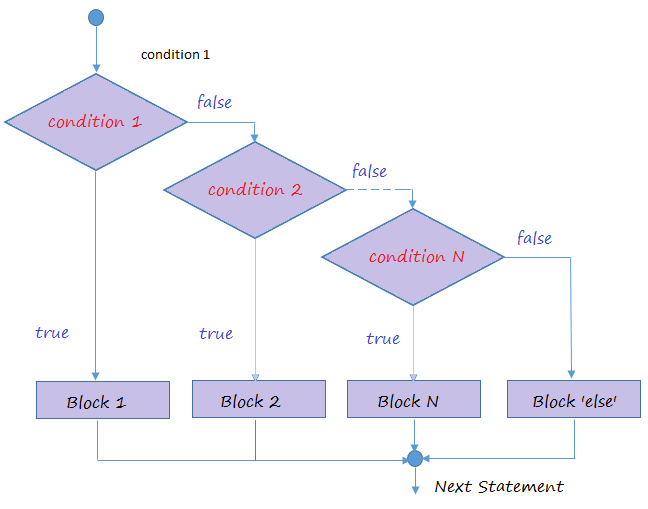Create ElseIfExample1 class: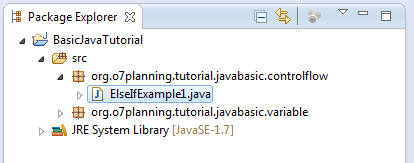ElseIfExample1.java
``````
package org.o7planning.tutorial.javabasic.controlflow;

public class ElseIfExample1 {

public static void main(String[] args) {

// Declaring a varible of type int (32 bit integer)
// Represent your test scores.
int score = 70;

System.out.println("Your score =" + score);

// If the score is less than 50
if (score < 50) {
System.out.println("You are not pass");
}
// Else if the score more than or equal to 50 and less than 80.
else if (score >= 50 && score < 80) {
System.out.println("You are pass");
}
// Remaining cases (that is greater than or equal to 80)
else {
System.out.println("You are pass, good student!");
}

}
}
``````
Results of running the class ElseIfExample1:
``````
You are pass
``````
Change the value of the variable "score" in the above example and rerun the ElseIfExample1 class:
``````
int score = 20;
``````
``````
You are not pass
``````

## 4- Boolean value

boolean is a data type, it only has two values true or false.
Create class BooleanExample:
BooleanExample.java
``````
package org.o7planning.tutorial.javabasic.controlflow;

public class BooleanExample {

public static void main(String[] args) {

// Declare a variable of type boolean
boolean value = true;

// If value is true
if (value == true) {
System.out.println("It's true");
}
// Else
else {
System.out.println("It's false");
}

if (value) {
System.out.println("It's true");
}
// Else
else {
System.out.println("It's false");
}
}
}
``````
``````
It's true
It's true
``````

## 5- Operators involved in conditional expression

This is a list of operators, commonly used in conditional expressions.
• > Greater Than
• < Less Than
• >= Greater Than or Equal To
• <= Less Than or Equal To
• && AND
• || OR
• == HAS A VALUE OF
• != Not Equal To
• ! NOT
Example:
ElseIfExample2.java
``````
package org.o7planning.tutorial.javabasic.controlflow;

public class ElseIfExample2 {

public static void main(String[] args) {

// Declare a variable of type int, represents your age.
int age = 20;

// Test if age less than or equal 17
if (age <= 17) {
System.out.println("You are 17 or younger");
}

// Test age equals 18
else if (age == 18) {
System.out.println("You are 18 year old");
}
// Test if age greater than 18 and less than 40
else if (age > 18 && age < 40) {
System.out.println("You are between 19 and 39");
}
// Remaining cases (Greater than or equal to 40)
else {
// Nested if statements
// Test age not equals 50.
if (age != 50) {
System.out.println("You are not 50 year old");
}

// Negative statements
if (!(age == 50)) {
System.out.println("You are not 50 year old");
}

// If age is 60 or 70
if (age == 60 || age == 70) {
System.out.println("You are 60 or 70 year old");
}

}

}
}
``````
Chạy ví dụ:
``````
You are between 19 and 39
``````
You can change the value of "age" and rerun ElseIfExample2 class and see the results

#### View more Tutorials:

Maybe you are interested

These are online courses outside the o7planning website that we introduced, which may include free or discounted courses.

•Serverless Architecture on Amazon Web Services
•Java Object-Oriented Programming : Build a Quiz Application
•Learn JavaScript for Web Development
•Google DART Programming HANDS-ON with PYTHON File Handling
•C# Unity® and Swift 4 Masterclass: Make Games and Apps
•Full Stack JavaScript: Learn Backbone.js, Node.js & MongoDB
•MySQL PHP Database Essentials add a Database to your site
•*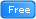* Java Database Connection: JDBC and MySQL
•Flutter Zero to Professional: cross platform App iOS/Android
•2D Game Development With HTML5 Canvas, JS - Tic Tac Toe Game
•Mastering Micro Services Using Java Spring Boot
•Introducing Dart
•Learn Partitioning in PostgreSQL from Scratch
•Create Android and iOS App using HTML, CSS and JS
•LEARNING PATH: SQL: Securing Data with SQL Server on Linux
•HTML5, CSS3 And JavaScript Fundamentals 2016
•Java JDBC with Oracle: Build a CRUD Application
•Backup and Restore Fundamentals in PostgreSQL DB - Level 1
•MySQL Made Simple For Beginners
•HTML5, CSS3 & Bootstrap - How to Create a Responsive Website
•Build Responsive Website with HTML5 and CSS3
•Understanding JDBC with PostgreSQL (A step by step guide)
•Complete Step By Step Java For Testers
•Build Responsive Website Using HTML5, CSS3, JS And Bootstrap